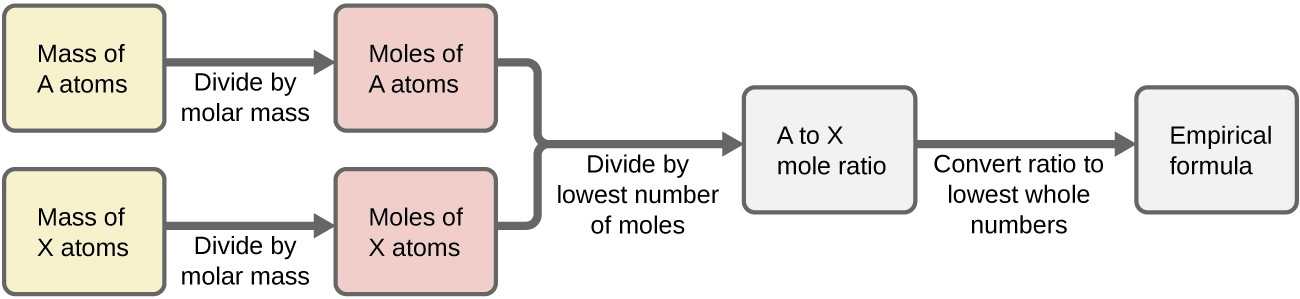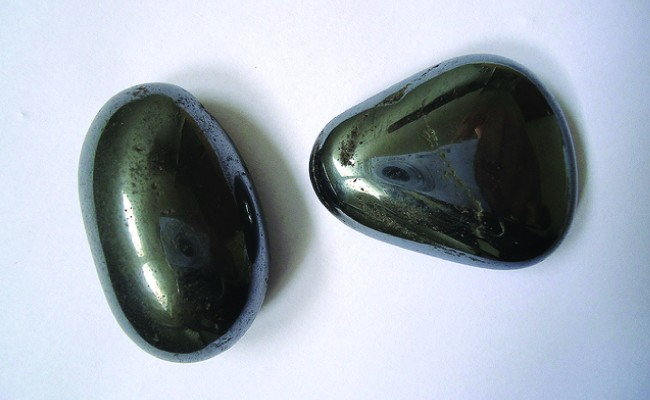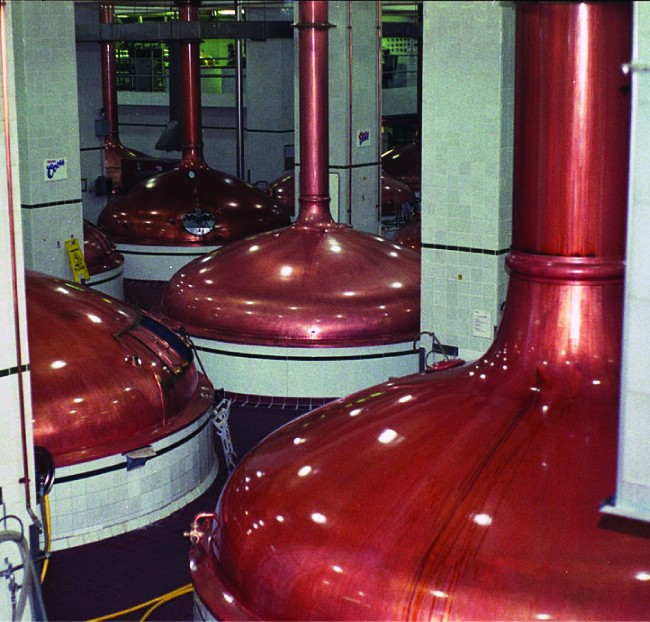## Determining Empirical and Molecular Formulas

### Learning Outcomes

• Compute the percent composition of a compound
• Determine the empirical formula of a compound
• Determine the molecular formula of a compound

In the previous section, we discussed the relationship between the bulk mass of a substance and the number of atoms or molecules it contains (moles). Given the chemical formula of the substance, we were able to determine the amount of the substance (moles) from its mass, and vice versa. But what if the chemical formula of a substance is unknown? In this section, we will explore how to apply these very same principles in order to derive the chemical formulas of unknown substances from experimental mass measurements.

## Percent Composition

The elemental makeup of a compound defines its chemical identity, and chemical formulas are the most succinct way of representing this elemental makeup. When a compound’s formula is unknown, measuring the mass of each of its constituent elements is often the first step in the process of determining the formula experimentally. The results of these measurements permit the calculation of the compound’s percent composition, defined as the percentage by mass of each element in the compound. For example, consider a gaseous compound composed solely of carbon and hydrogen. The percent composition of this compound could be represented as follows:

$\%\text{H}=\dfrac{\text{mass H}}{\text{mass compound}}\times 100\%$

$\%\text{C}=\dfrac{\text{mass C}}{\text{mass compound}}\times 100\%$

If analysis of a 10.0-g sample of this gas showed it to contain 2.5 g H and 7.5 g C, the percent composition would be calculated to be 25% H and 75% C:

$\%\text{H}=\dfrac{2.5\text{g H}}{10.0\text{g compound}}\times 100\%=25\%$

$\%\text{C}=\dfrac{7.5\text{g C}}{10.0\text{g compound}}\times 100\%=75\%$

### Example 1: Calculation of Percent Composition

Analysis of a 12.04-g sample of a liquid compound composed of carbon, hydrogen, and nitrogen showed it to contain 7.34 g C, 1.85 g H, and 2.85 g N. What is the percent composition of this compound?

A 24.81-g sample of a gaseous compound containing only carbon, oxygen, and chlorine is determined to contain 3.01 g C, 4.00 g O, and 17.81 g Cl. What is this compound’s percent composition?

### Determining Percent Composition from Molecular or Empirical Formulas

Percent composition is also useful for evaluating the relative abundance of a given element in different compounds of known formulas. As one example, consider the common nitrogen-containing fertilizers ammonia (NH3), ammonium nitrate (NH4NO3), and urea (CH4N2O). The element nitrogen is the active ingredient for agricultural purposes, so the mass percentage of nitrogen in the compound is a practical and economic concern for consumers choosing among these fertilizers. For these sorts of applications, the percent composition of a compound is easily derived from its formula mass and the atomic masses of its constituent elements. A molecule of NH3 contains one N atom weighing 14.01 amu and three H atoms weighing a total of (3 × 1.008 amu) = 3.024 amu The formula mass of ammonia is therefore (14.01 amu + 3.024 amu) = 17.03 amu, and its percent composition is:

$\begin{array}{c}\\ \%\text{N}=\dfrac{14.01\text{ amu N}}{17.03\text{ amu }{\text{ NH}}_{3}}\times 100\%=82.27\%\\ \%\text{H}=\dfrac{3.024\text{ amu N}}{17.03\text{ amu }{\text{ NH}}_{3}}\times 100\%=17.76\%\end{array}$

This same approach may be taken considering a pair of molecules, a dozen molecules, or a mole of molecules, etc. The latter amount is most convenient and would simply involve the use of molar masses instead of atomic and formula masses, as demonstrated in the example problem below. As long as we know the chemical formula of the substance in question, we can easily derive percent composition from the formula mass or molar mass.

### Example 2: Determining Percent Composition from a Molecular Formula

Aspirin is a compound with the molecular formula C9H8O4. What is its percent composition?

To three significant digits, what is the mass percentage of iron in the compound Fe2O3?

### Try It

1. Calculate the following to four significant figures:
1. the percent composition of ammonia, NH3
2. the percent composition of photographic “hypo,” Na2S2O3
3. the percent of calcium ion in Ca3(PO4)2
2. Determine the following to four significant figures:
1. the percent composition of hydrazoic acid, HN3
2. the percent composition of TNT, C6H2(CH3)(NO2)3
3. the percent of SO42– in Al2(SO4)3
3. Determine the percent ammonia, NH3, in Co(NH3)6Cl3, to three significant figures.
4. Determine the percent water in CuSO4∙5H2O to three significant figures.

## Determination of Empirical Formulas

As previously mentioned, the most common approach to determining a compound’s chemical formula is to first measure the masses of its constituent elements. However, we must keep in mind that chemical formulas represent the relative numbers, not masses, of atoms in the substance. Therefore, any experimentally derived data involving mass must be used to derive the corresponding numbers of atoms in the compound. To accomplish this, we can use molar masses to convert the mass of each element to a number of moles. We then consider the moles of each element relative to each other, converting these numbers into a whole-number ratio that can be used to derive the empirical formula of the substance. Consider a sample of compound determined to contain 1.71 g C and 0.287 g H. The corresponding numbers of atoms (in moles) are:

$\begin{array}{l}1.17\text{g C}\times \dfrac{1\text{mol C}}{12.01\text{g C}}=0.142\text{mol C}\\ 0.287\text{g H}\times \dfrac{1\text{mol H}}{1.008\text{g H}}=0.284\text{mol H}\end{array}$

Thus, we can accurately represent this compound with the formula C0.142H0.248. Per accepted convention, formulas contain whole-number subscripts, which can be achieved by dividing each subscript by the smaller subscript:

$\displaystyle{\text{C}}_{\frac{0.142}{0.142}}{\text{H}}_{\frac{0.248}{0.142}}\text{ or }{\text{CH}}_{2}$

(Recall that subscripts of “1” are not written but rather assumed if no other number is present.)

The empirical formula for this compound is thus CH2. This may or not be the compound’s molecular formula as well; however, we would need additional information to make that determination (as discussed later in this section).

Consider as another example a sample of compound determined to contain 5.31 g Cl and 8.40 g O. Following the same approach yields a tentative empirical formula of:

${\text{C1}}_{0.150}{\text{O}}_{0.525}={\text{Cl}}_{\frac{0.150}{0.150}}{\text{O}}_{\frac{0.525}{0.150}}={\text{ClO}}_{3.5}$

In this case, dividing by the smallest subscript still leaves us with a decimal subscript in the empirical formula. To convert this into a whole number, we must multiply each of the subscripts by two, retaining the same atom ratio and yielding Cl2O7 as the final empirical formula.

In summary, empirical formulas are derived from experimentally measured element masses by:

1. Deriving the number of moles of each element from its mass
2. Dividing each element’s molar amount by the smallest molar amount to yield subscripts for a tentative empirical formula
3. Multiplying all coefficients by an integer, if necessary, to ensure that the smallest whole-number ratio of subscripts is obtained

Figure 1 outlines this procedure in flow chart fashion for a substance containing elements A and X.Figure 1. The empirical formula of a compound can be derived from the masses of all elements in the sample.

### Example 3: Determining a Compound’s Empirical Formula from the Masses of Its Elements

A sample of the black mineral hematite (Figure 2), an oxide of iron found in many iron ores, contains 34.97 g of iron and 15.03 g of oxygen. What is the empirical formula of hematite?Figure 2. Hematite is an iron oxide that is used in jewelry. (credit: Mauro Cateb)

What is the empirical formula of a compound if a sample contains 0.130 g of nitrogen and 0.370 g of oxygen?

For additional worked examples illustrating the derivation of empirical formulas, watch the brief video clip below.

### Deriving Empirical Formulas from Percent Composition

Finally, with regard to deriving empirical formulas, consider instances in which a compound’s percent composition is available rather than the absolute masses of the compound’s constituent elements. In such cases, the percent composition can be used to calculate the masses of elements present in any convenient mass of compound; these masses can then be used to derive the empirical formula in the usual fashion.

### Example 4: Determining an Empirical Formula from Percent Composition

The bacterial fermentation of grain to produce ethanol forms a gas with a percent composition of 27.29% C and 72.71% O (Figure 3). What is the empirical formula for this gas?Figure 3. An oxide of carbon is removed from these fermentation tanks through the large copper pipes at the top. (credit: “Dual Freq”/Wikipedia)

What is the empirical formula of a compound containing 40.0% C, 6.71% H, and 53.28% O?

## Derivation of Molecular Formulas

Recall that empirical formulas are symbols representing the relative numbers of a compound’s elements. Determining the absolute numbers of atoms that compose a single molecule of a covalent compound requires knowledge of both its empirical formula and its molecular mass or molar mass. These quantities may be determined experimentally by various measurement techniques. Molecular mass, for example, is often derived from the mass spectrum of the compound (see discussion of this technique in the previous module on atoms and molecules). Molar mass can be measured by a number of experimental methods, many of which will be introduced later.

Molecular formulas are derived by comparing the compound’s molecular or molar mass to its empirical formula mass. As the name suggests, an empirical formula mass is the sum of the average atomic masses of all the atoms represented in an empirical formula. If we know the molecular (or molar) mass of the substance, we can divide this by the empirical formula mass in order to identify the number of empirical formula units per molecule, ($n$):

$\displaystyle\dfrac{\text{molecular or molar mass}\left(\text{amu or}\dfrac{\text{g}}{\text{mol}}\right)}{\text{empirical formula mass}\left(\text{amu or}\dfrac{\text{g}}{\text{mol}}\right)}=n\text{ formula units/molecule}$

The molecular formula is then obtained by multiplying each subscript in the empirical formula by $n$, as shown below for the generic empirical formula $A_{x}B_{y}$:

${{\text{(A}}_{\text{x}}{\text{B}}_{\text{y}}\text{)}}_{\text{n}}={\text{A}}_{\text{nx}}{\text{B}}_{\text{nx}}$

For example, consider a covalent compound whose empirical formula is determined to be CH2O. The empirical formula mass for this compound is approximately 30 amu (the sum of 12 amu for one C atom, 2 amu for two H atoms, and 16 amu for one O atom). If the compound’s molecular mass is determined to be 180 amu, this indicates that molecules of this compound contain six times the number of atoms represented in the empirical formula:

$\dfrac{180\dfrac{\text{amu}}{\text{molecule}}}{30\dfrac{\text{amu}}{\text{formula unit}}}=6\dfrac{\text{formula units}}{\text{molecule}}$

Molecules of this compound are then represented by molecular formulas whose subscripts are six times greater than those in the empirical formula: ${\text{(CH}}_{\text{2}}{\text{O)}}_{\text{6}}={\text{C}}_{\text{6}}{\text{H}}_{\text{12}}{\text{O}}_{\text{6}}$

Note that this same approach may be used when the molar mass (g/mol) instead of the molecular mass (amu) is used. In this case, we are merely considering one mole of empirical formula units and molecules, as opposed to single units and molecules.

### Example 5: Determination of the Molecular Formula for Nicotine

Nicotine, an alkaloid in the nightshade family of plants that is mainly responsible for the addictive nature of cigarettes, contains 74.02% C, 8.710% H, and 17.27% N. If 40.57 g of nicotine contains 0.2500 mol nicotine, what is the molecular formula?

What is the molecular formula of a compound with a percent composition of 49.47% C, 5.201% H, 28.84% N, and 16.48% O, and a molecular mass of 194.2 amu?

### Key Concepts and Summary

The chemical identity of a substance is defined by the types and relative numbers of atoms composing its fundamental entities (molecules in the case of covalent compounds, ions in the case of ionic compounds). A compound’s percent composition provides the mass percentage of each element in the compound, and it is often experimentally determined and used to derive the compound’s empirical formula. The empirical formula mass of a covalent compound may be compared to the compound’s molecular or molar mass to derive a molecular formula.

#### Key Equations

• $\%\text{ X}=\dfrac{\text{mass X}}{\text{mass compound}}\times 100\%$
• $\dfrac{\text{molecular or molar mass}\left(\text{amu or}\dfrac{\text{g}}{\text{mol}}\right)}{\text{empirical formula mass}\left(\text{amu or}\dfrac{\text{g}}{\text{mol}}\right)}=n\text{ formula units/molecule}$
• $(A_{x}B_{y})_{n}=A_{nx}B_{ny}$

### Try It

1. What information do we need to determine the molecular formula of a compound from the empirical formula?
2. Determine the empirical formulas for compounds with the following percent compositions:
1. 15.8% carbon and 84.2% sulfur
2. 40.0% carbon, 6.7% hydrogen, and 53.3% oxygen
3. Determine the empirical formulas for compounds with the following percent compositions:
1. 43.6% phosphorus and 56.4% oxygen
2. 28.7% K, 1.5% H, 22.8% P, and 47.0% O
4. Polymers are large molecules composed of simple units repeated many times. Thus, they often have relatively simple empirical formulas. Calculate the empirical formulas of the following polymers:
1. Lucite (Plexiglas); 59.9% C, 8.06% H, 32.0% O
2. Saran; 24.8% C, 2.0% H, 73.1% Cl
3. polyethylene; 86% C, 14% H
4. polystyrene; 92.3% C, 7.7% H
5. Orlon; 67.9% C, 5.70% H, 26.4% N
5. A compound of carbon and hydrogen contains 92.3% C and has a molar mass of 78.1 g/mol. What is its molecular formula?
6. Dichloroethane, a compound that is often used for dry cleaning, contains carbon, hydrogen, and chlorine. It has a molar mass of 99 g/mol. Analysis of a sample shows that it contains 24.3% carbon and 4.1% hydrogen. What is its molecular formula?
7. Determine the empirical and molecular formula for chrysotile asbestos. Chrysotile has the following percent composition: 28.03% Mg, 21.60% Si, 1.16% H, and 49.21% O. The molar mass for chrysotile is 520.8 g/mol.
8. A major textile dye manufacturer developed a new yellow dye. The dye has a percent composition of 75.95% C, 17.72% N, and 6.33% H by mass with a molar mass of about 240 g/mol. Determine the molecular formula of the dye.

## Glossary

empirical formula mass: sum of average atomic masses for all atoms represented in an empirical formula

percent composition: percentage by mass of the various elements in a compound